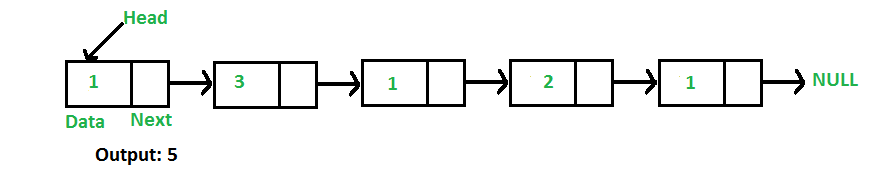# Java Program For Finding Length Of A Linked List

• Last Updated : 27 Dec, 2021

Write a function to count the number of nodes in a given singly linked list.For example, the function should return 5 for linked list 1->3->1->2->1.

Iterative Solution:

```1) Initialize count as 0
2) Initialize a node pointer, current = head.
3) Do following while current is not NULL
a) current = current -> next
b) count++;
4) Return count ```

Following is the Iterative implementation of the above algorithm to find the count of nodes in a given singly linked list.

## Java

 `// Java program to count number of ``// nodes in a linked list`` ` `// Linked list Node``class` `Node``{``    ``int` `data;``    ``Node next;``    ``Node(``int` `d)  { data = d;  next = ``null``; }``}`` ` `// Linked List class``class` `LinkedList``{``    ``// Head of list``    ``Node head;  `` ` `    ``// Inserts a new Node at front ``    ``// of the list.``    ``public` `void` `push(``int` `new_data)``    ``{``        ``/* 1 & 2: Allocate the Node &``                  ``Put in the data*/``        ``Node new_node = ``new` `Node(new_data);`` ` `        ``// 3. Make next of new Node as head ``        ``new_node.next = head;`` ` `        ``// 4. Move the head to point to new Node ``        ``head = new_node;``    ``}`` ` `    ``// Returns count of nodes in linked list``    ``public` `int` `getCount()``    ``{``        ``Node temp = head;``        ``int` `count = ``0``;``        ``while` `(temp != ``null``)``        ``{``            ``count++;``            ``temp = temp.next;``        ``}``        ``return` `count;``    ``}`` ` `    ``// Driver code``    ``public` `static` `void` `main(String[] args)``    ``{``        ``// Start with the empty list ``        ``LinkedList llist = ``new` `LinkedList();``        ``llist.push(``1``);``        ``llist.push(``3``);``        ``llist.push(``1``);``        ``llist.push(``2``);``        ``llist.push(``1``);`` ` `        ``System.out.println(``"Count of nodes is "` `+``                            ``llist.getCount());``    ``}``}`

Output:

`count of nodes is 5`

Recursive Solution:

```int getCount(head)
1) If head is NULL, return 0.
2) Else return 1 + getCount(head->next) ```

Following is the Recursive implementation of the above algorithm to find the count of nodes in a given singly linked list.

## Java

 `// Recursive Java program to count number ``// of nodes in a linked list`` ` `// Linked list Node``class` `Node``{``    ``int` `data;``    ``Node next;``    ``Node(``int` `d)  ``    ``{  ``        ``data = d;  ``        ``next = ``null``; ``    ``}``}`` ` `// Linked List class``class` `LinkedList``{``     ` `    ``Node head;  `` ` `    ``// Inserts a new Node at front of``    ``// the list. ``    ``public` `void` `push(``int` `new_data)``    ``{``        ``/* 1 & 2: Allocate the Node &``                  ``Put in the data*/``        ``Node new_node = ``new` `Node(new_data);`` ` `        ``// 3. Make next of new Node as head ``        ``new_node.next = head;`` ` `        ``// 4. Move the head to point to new Node``        ``head = new_node;``    ``}`` ` `    ``// Returns count of nodes in linked list``    ``public` `int` `getCountRec(Node node)``    ``{``        ``// Base case``        ``if` `(node == ``null``)``            ``return` `0``;`` ` `        ``// Count is this node plus rest ``        ``// of the list``        ``return` `1` `+ getCountRec(node.next);``    ``}`` ` `    ``// Wrapper over getCountRec() ``    ``public` `int` `getCount()``    ``{``        ``return` `getCountRec(head);``    ``}`` ` `    ``// Driver code``    ``public` `static` `void` `main(String[] args)``    ``{``        ``// Start with the empty list``        ``LinkedList llist = ``new` `LinkedList();``        ``llist.push(``1``);``        ``llist.push(``3``);``        ``llist.push(``1``);``        ``llist.push(``2``);``        ``llist.push(``1``);`` ` `        ``System.out.println(``"Count of nodes is "` `+``                            ``llist.getCount());``    ``}``}`

Output:

`Count of nodes is 5`

Please refer complete article on Find Length of a Linked List (Iterative and Recursive) for more details!

My Personal Notes arrow_drop_up